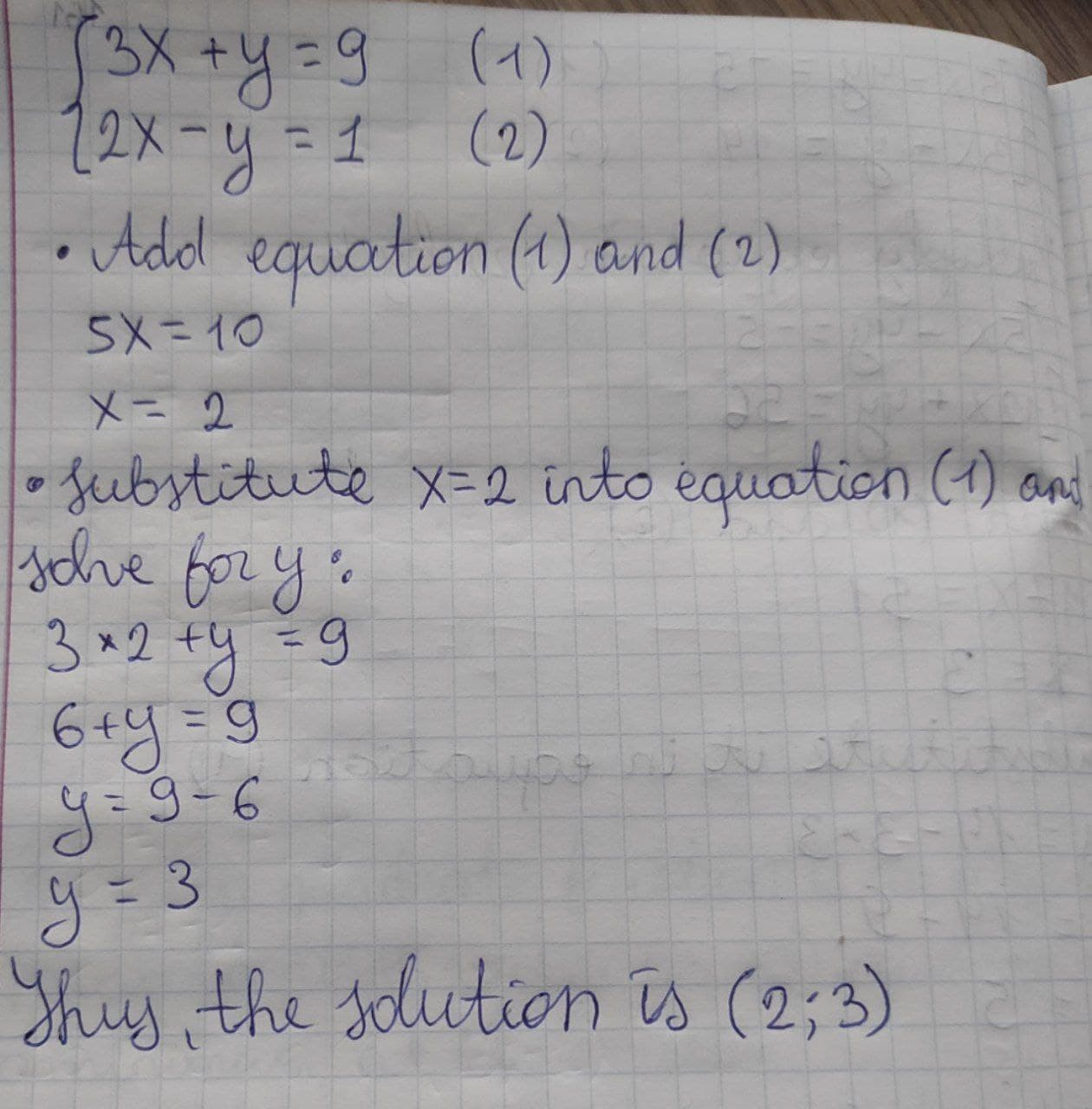# Solve the system: {(3x,+,y,=,9),(2x,-,y,=,1):}Question
Systems of equationsSolve the system: {(3x,+,y,=,9),(2x,-,y,=,1):}2020-12-29

{(3x,+,y,=,9),(2x,-,y,=,1):} Add equation (1) and (2): 5x = 10 x = 2 Substitute x = 2 into equation (1) and solve for y: 3 xx 2 + y = 9 6 + y = 9 y = 9 – 6 y = 3 Thus, the solution is (2,3)### Relevant QuestionsSolve the system of equations. {(x,+,4y,=,-2),(-2x,+12y,=,9):}Solve the system: {(-3x,+,y,=,2),(9x,-,3y,=,-6):}Solve the system: {(-2x,+,y,=,5),(-6x,+,3y,=,21):}Please, solve the system of equations: {(5x,+,y,=,14),(2x,+,y,=,5):}Solve {(x,+,2y,=,9),(y,=,-2x):}What is the solution of the system of equations?
y = 2x - 3
5x + y = 11
A (2, 1)
B (1, 2)
C (3, -4)
D (1, -1)Solve {(2x,+,3y,=,-1),(6x,+,3y,=,-9):}x-y+2x=3
2x+z=1
3x+2y+z=4$$y = x^{2} + 3x − 7$$
$$3x − y = −2$$